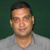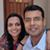D

#### Understanding Newton's third law

137 viewed last edited 2 years agoAnonymous
1
I understand that if an object is floating on water then the action is the object's weight and the reaction is the buoyant force holding the object up. What is the action and reaction when the object is sinking and not floating? Which one is the action and which one is the reaction? Obviously, the buoyant force cannot be the reaction as it would be less than the object's weight.Anand Kannan
6

The language of "action" and "reaction" is incorrectly applied by the question. Weight is force exerted by gravity on the body - the action. The body pulls the earth back with the same force - there is the reaction. The water exerts pressure on the surface of the body - that is the action. The body exerts pressure on the water - that is the reaction. The net motion of the body depends on the net effect of the weight and the pressure from the water (aka buoyancy force). This follows newton's second law where it says the rate of change of momentum equal to the net force is produced. The action-reaction does not predict the dynamics of the body. It is third law. first and second laws are needed to understand the resultant change in motion. (going up or down).Mahesh Godavarti
4

There is an error in your understanding.

Remember that action and reaction have to arise from the same pair of interacting objects.

In the example that you stated where the object is floating on water, the object's weight and the buoyant force are NOT the action and reaction. The object's weight arises from its interaction with the Earth. Therefore, the correct reaction to the object's weight is gravity pulling the Earth towards the object.

The correct action to the buoyant force (that we have identified as the reaction) is the normal force pushing down on water. If the object is floating then it so happens that the weight and the buoyant force turn out to be equal to each other in magnitude and opposite in direction. Note that this does not mean that they are an action and reaction pair.

The interacting forces remain the same even when the object is sinking.

1. The normal force pushing down on water. The buoyant force pushing up on the object.
2. The object's weight. The gravitation force pulling the Earth towards the object.

It so happens that if the object is sinking then the two forces acting on the object are not equal. However, the interacting forces are equal to each other.

We can also add another set of interacting forces between the object and water:

1. The drag from water on the object as it sinks. And the thrust from the object on water as it sinks.Craig Young
2
Mahesh - I like your explanation. Very clear. Although I wouldn't refer to the downward force on the water from the object as "thrust"; it's still buoyancy just the other half of the force interaction. Thrust would be a fish or squid pushing back on the water and the water pushing the fish/squid forward. Thrust is a push forward on the object in the direction of motion from the fluid. BTW, this whole thread demonstrates why I avoid "action" and "reaction" forces with my students - too much possibility for confusion. I always go with "when object A is pushing/pulling in object B, then object B is also.pushing/pulling on object A in the opposite direction".4

Action and reaction always act on different bodies. Since gravity and buoyant force act on the same "object", they cannot be action-reaction pairs.

More detail:

Bouyant force is an action, not a reaction. There is a reaction force from the object back onto the surface of water, which is equal and opposite to the buoyant force. This is independent of whether the object sinks or floats.

Gravity is another action, caused by the presence of a large body (earth), which pulls both the "object" and the "water" towards it. The reaction force to gravity is gravity itself - both the "object" and "water" pull the earth towards them with equal force!

If gravity > buoyant force, the object sinks. If buoyant force > gravity, the object floats until sufficient parts of it are out of the water, till buoyant force = gravity.Prem Kumar
3

Hi, that's an interesting question. But it also requires a bit of qualification.

Generally speaking, it is better to qualify the terms ``action" and ``reaction" by being more specific e.g. ``action of A on B" and ``reaction of B on A".

Note that buoyancy is a phenomenon that is exhibited by objects with extent i.e. with a length, breadth and height. So let's take a cube for simplicity. If the mass is light enough so the cube floats, then the ``action'' is the force exerted by the bottom surface of the cube on the water layer below it, and is equal to the weight of the cube. The reaction of the water layer on the bottom surface is equal and opposite.

Now let's take the case of the cube heavy enough so it is just sinking i.e slowly . "Slowly" is important here to think in terms of hydrostatics.. Hydrodynamics will complicate the picture. The bottom surface of the cube exerts a force on the layer of water just below it (action). This is equal to force exerted by the layer of water on the bottom surface of the cube which must be equal to the pressure times area at the bottom of the cube. This has to be the case for the water layers to not move much.

Similarly on the top surface of the cube, the water on the top exerts a (lower) pressure proportional to the height of the water column above on the top surface of the cube. The force of this on the top surface of the cube is pressure above times area of the top surface of the cube. The cube exerts an equal and opposite reaction (in the upward direction) on the water layer adjacent to the top of the cube.

So there is no violation of the action-reaction principle, just that action and reaction effects are taking place differently and between different objects (different portions of the fluid and of the cube).

Note that independent of all of this the cube pulls the earth (action) by the force of gravity whose magnitude is the weight of the cube and the earth pulls on the cube with a force of the same magnitude but opposite sign.Krishna
1

Hai

Your way of thinking is good. What you said is correct.

Newton's third law

Action = reaction (Weight = Buoyant force) Then the object Float on the water

Action \neq reaction (Weight \neq Buoyant force) Then the object sink in the water.

Here weight is high compared to the buoyant force (buoyant force is also there but it is decreasing continually. When the object reaches the bottom then is it become zero.)

Buoyancy:

Buoyancy is the upward force that an object feels from the water and when compared to the weight of the object, it is what makes an object float, sink, or remain neutrally buoyant in the water.

When an object floats, the upward buoyant force exerted by the water is greater than the downward force of the weight of the object.

or

If an object’s density is less than water’s density it will float.

When an object sinks, the weight of the object is greater than the upward buoyant force exerted by the water

Object neither sinks nor floats, then the weight of the object is equal to the upward buoyant force exerted by the water.Mahesh Godavarti
1
What would be the equal and opposite reaction here? A clarification for that would be good.